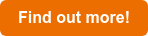# Predictive Analytics with Vertica## In-SQL machine learning

Vertica is a clustered analytical database that handles large, fast-growing volumes of data with ease and provides lightning fast query performance. Apart from that it also has in-database machine learning which we’ll be taking a look at in this blogpost!

As an avid fan of SpaceX and all things Tesla I took to the internet to find a fun dataset and landed on one of the go-to websites for machine learning, kaggle. The dataset itself is scraped from SpaceX and NASA containing the outcome of SpaceX missions from 2006 to the present. It’s a rather small sample to train a model on but enough for the purposes of this post. This won't be about how good Vertica is, but rather how easy it is to apply some basic machine learning with it. You can use your favorite tool to load the data into Vertica; I used PDI and created a database named spacex_flights.

Let's start by performing some queries to explore the data.

Flights per year:

 => select date_part('YEAR', launch_date), count(*) from spacex_flights group by 1 order by 1;Flights success per payload mass in kg:

 => select payload_mass_kg, case when mission_outcome = 1 then 'success' else 'failure' end from spacex_flights where payload_mass_kg is not null order by 1 desc;Mission failure versus success:

 => select count(nullif(mission_outcome, 1)) as failure, count(nullif(mission_outcome, 0)) as success from spacex_flights;## Moving to machine learning

Two things are worth investigating here: predicting the number of flights and how payload weight influences mission success.
We’ll use two regression algorithms to build a model for each.

Predicting flights based on the year:

Create a 'training' table from the source table, this can be a selection with aggregates for the model to train on:

 => create table training as (select date_part('YEAR', launch_date) as year, count(*) as missions from spacex_flights group by 1 order by 2);

We select the year and a sum of the number of missions.

Create a linear regression model named model_flights based on the previously created 'training' table using year

 => select linear_reg('model_flights','training','missions','year' using parameters optimizer='BFGS');

The model is trained by using missions as a response column (the outcome) and year as a predictor.
We use linear regression to predict continuous numerical outcomes based on a linear relationship.

Show a summary of the model using the following command:

 => select get_model_summary(using parameters model_name='model_flights');

Make a comparison of the existing values next to the model's predictions:

 => select year, missions, predict_linear_reg(year using parameters model_name='model_flights') as pred from training ;

Here we can estimate how well our model works, but what happens when we have a larger dataset? Calculating the mean squared error will help with this.

Create a prediction table:

 => create table predictionResults as (select year, missions, predict_linear_reg(year using parameters model_name='model_flights') as pred from training );

As a way to check our model proficiency we'll calculate the mean squared error (MSE), MSE returns the average of squared differences between the original values and the model's:

 => select MSE(obs::float, prediction::float) over() from (select missions as obs, pred as prediction from predictionResults) as prediction_output;

Now for new predictions, create a test table with new values to see how the model handles new data:

 => create table test (year int);=> insert into test values (2006);=> insert into test values (2018); => select year, predict_linear_reg(year using parameters model_name='model_flights') as predicted_missions from test;Predicting mission success based on payload mass:

Again we create a 'training' table from the source table using payload mass and the mission success:

 => create table training as (select coalesce(payload_mass_kg, (select avg(payload_mass_kg) from spacex_flights )) as payload_mass_kg, case when mission_outcome = coalesce(landing_outcome, 0) then 1 else 0 end as mission_success from spacex_flights);

We'll use payload mass (and an average if it is empty).
Let's also consider the mission a success when both the 'mission_outcome' and 'landing_outcome' are a success.

Create a logistic model using the new column 'mission_success' and

 => select logistic_reg('modelSuccess','training','mission_success','payload_mass_kg' using parameters optimizer='BFGS');

Here we use 'payload_mass_kg' and 'mission_success' to train a logistic regression model modelSuccess. We use logistic regression for predicting binary outcomes.
For instance, was the mission succesful yes or no?

Get the model summary:

 => select get_model_summary(using parameters model_name='modelSuccess');

Show a comparison like before:

 => select payload_mass_kg, mission_success, predict_logistic_reg(payload_mass_kg using parameters model_name='modelSuccess') as pred from training ;

To evaluate the proficiency of this model we'll use a confusion matrix evaluation function:

 => create table predictionResults as (select payload_mass_kg, mission_success, predict_logistic_reg(payload_mass_kg using parameters model_name='modelSuccess') as pred from training ); => select confusion_matrix(obs::int, prediction::int using parameters num_classes=2) over() from (select mission_success as obs, pred as prediction from predictionResults) as prediction_output;or:Just like before, we create a table with prediction results. We then apply the confusion matrix function which shows us that the model correctly predicted 4 missions to be succesful and 15 missions to be unsuccesful. It also tells us the model incorrectly predicted 6 missions to be successful and 16 to be unsuccesful.

## Conclusion

Vertica offers an easy way to execute in-database predictive analytics which makes it a very neat addition to do some data exploration (especially on larger datasets), with the big advantage that we can keep the data within the database and only use SQL.

Can't wait to find out more?